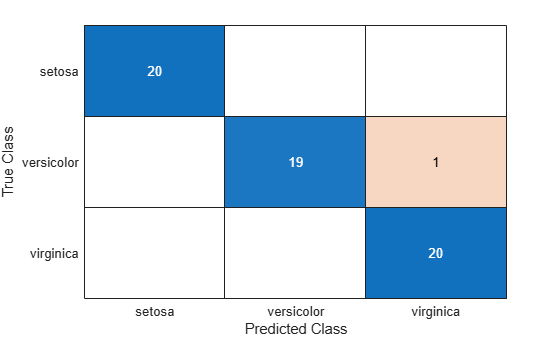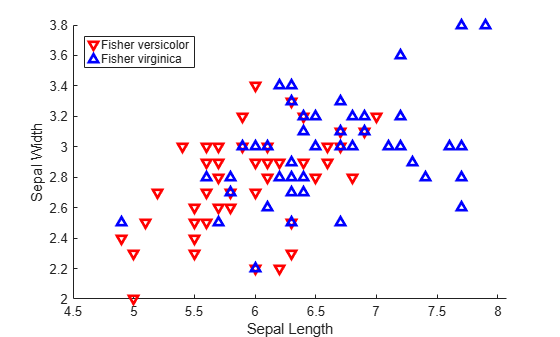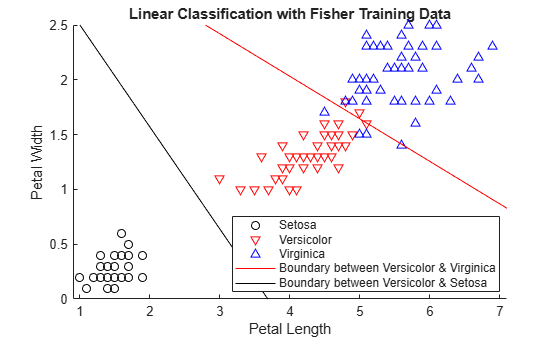# classify

Classify observations using discriminant analysis

## Syntax

``class = classify(sample,training,group)``
``class = classify(sample,training,group,type,prior)``
``[class,err,posterior,logp,coeff] = classify(___)``

## Description

example

``` Note`fitcdiscr` and `predict` are recommended over `classify` for training a discriminant analysis classifier and predicting labels. `fitcdiscr` supports cross-validation and hyperparameter optimization, and does not require you to fit the classifier every time you make a new prediction or change prior probabilities. `class = classify(sample,training,group)` classifies each row of the data in `sample` into one of the groups to which the data in `training` belongs. The groups for `training` are specified by `group`. The function returns `class`, which contains the assigned groups for each row of `sample`.```
````class = classify(sample,training,group,type,prior)` also specifies the `type` of discriminant function, and `prior` probabilities for each group.```

example

````[class,err,posterior,logp,coeff] = classify(___)` also returns the apparent error rate (`err`), posterior probabilities for training observations (`posterior`), logarithm of the unconditional probability density for sample observations (`logp`), and coefficients of the boundary curves (`coeff`), using any of the input argument combinations in previous syntaxes.```

## Examples

collapse all

Load the `fisheriris` data set. Create `group` as a cell array of character vectors that contains the iris species.

```load fisheriris group = species;```

The `meas` matrix contains four petal measurements for 150 irises. Randomly partition observations into a training set (`trainingData`) and a sample set (`sampleData`) with stratification, using the group information in `group`. Specify a 40% holdout sample for `sampleData`.

```rng('default') % For reproducibility cv = cvpartition(group,'HoldOut',0.40); trainInds = training(cv); sampleInds = test(cv); trainingData = meas(trainInds,:); sampleData = meas(sampleInds,:);```

Classify `sampleData` using linear discriminant analysis, and create a confusion chart from the true labels in `group` and the predicted labels in `class`.

```class = classify(sampleData,trainingData,group(trainInds)); cm = confusionchart(group(sampleInds),class);```The `classify` function misclassifies one versicolor iris as virginica in the sample data set.

Classify the data points in a grid of measurements (sample data) by using quadratic discriminant analysis. Then, visualize the sample data, training data, and decision boundary.

Load the `fisheriris` data set. Create `group` as a cell array of character vectors that contains the iris species.

```load fisheriris group = species(51:end);```

Plot the sepal length (`SL`) and width (`SW`) measurements for the iris versicolor and virginica species.

```SL = meas(51:end,1); SW = meas(51:end,2); h1 = gscatter(SL,SW,group,'rb','v^',[],'off'); h1(1).LineWidth = 2; h1(2).LineWidth = 2; legend('Fisher versicolor','Fisher virginica','Location','NW') xlabel('Sepal Length') ylabel('Sepal Width')```Create `sampleData` as a numeric matrix that contains a grid of measurements. Create `trainingData` as a numeric matrix that contains the sepal length and width measurements for the iris versicolor and virginica species.

```[X,Y] = meshgrid(linspace(4.5,8),linspace(2,4)); X = X(:); Y = Y(:); sampleData = [X Y]; trainingData = [SL SW];```

Classify `sampleData` using quadratic discriminant analysis.

`[C,err,posterior,logp,coeff] = classify(sampleData,trainingData,group,'quadratic');`

Retrieve the coefficients `K`, `L`, and `M` for the quadratic boundary between the two classes.

```K = coeff(1,2).const; L = coeff(1,2).linear; Q = coeff(1,2).quadratic;```

The curve that separates the two classes is defined by this equation:

`$\mathit{K}+\left[\begin{array}{cc}{\mathit{x}}_{1}& {\mathit{x}}_{2}\end{array}\right]\mathit{L}+\left[\begin{array}{cc}{\mathit{x}}_{1}& {\mathit{x}}_{2}\end{array}\right]\mathit{Q}\left[\begin{array}{c}{\mathit{x}}_{1}\\ {\mathit{x}}_{2}\end{array}\right]=0$`

Visualize the discriminant classification.

```hold on h2 = gscatter(X,Y,C,'rb','.',1,'off'); f = @(x,y) K + L(1)*x + L(2)*y + Q(1,1)*x.*x + (Q(1,2)+Q(2,1))*x.*y + Q(2,2)*y.*y; h3 = fimplicit(f,[4.5 8 2 4]); h3.Color = 'm'; h3.LineWidth = 2; h3.DisplayName = 'Decision Boundary'; hold off axis tight xlabel('Sepal Length') ylabel('Sepal Width') title('Classification with Fisher Training Data')```Partition a data set into sample and training data, and classify the sample data using linear discriminant analysis. Then, visualize the decision boundaries.

Load the `fisheriris` data set. Create `group` as a cell array of character vectors that contains the iris species. Create `PL` and `PW` as numeric vectors that contain the petal length and width measurements, respectively.

```load fisheriris group = species; PL = meas(:,3); PW = meas(:,4);```

Plot the sepal length (`PL`) and width (`PW`) measurements for the iris setosa, versicolor, and virginica species.

```h1 = gscatter(PL,PW,species,'krb','ov^',[],'off'); legend('Setosa','Versicolor','Virginica','Location','best') xlabel('Petal Length') ylabel('Petal Width')```Randomly partition observations into a training set (`trainingData`) and a sample set (`sampleData`) with stratification, using the group information in `group`. Specify a 10% holdout sample for `sampleData`.

```rng('default') % For reproducibility cv = cvpartition(group,'HoldOut',0.10); trainInds = training(cv); sampleInds = test(cv); trainingData = [PL(trainInds) PW(trainInds)]; sampleData = [PL(sampleInds) PW(sampleInds)];```

Classify `sampleData` using linear discriminant analysis.

`[class,err,posterior,logp,coeff] = classify(sampleData,trainingData,group(trainInds));`

Retrieve the coefficients `K` and `L` for the linear boundary between the second and third classes.

```K = coeff(2,3).const; L = coeff(2,3).linear;```

The line that separates the second and third classes is defined by the equation $\text{\hspace{0.17em}}\mathit{K}+\left[\begin{array}{cc}{\mathit{x}}_{1}& {\mathit{x}}_{2}\end{array}\right]\mathit{L}=0$. Plot the boundary line between the second and third classes.

```f = @(x1,x2) K + L(1)*x1 + L(2)*x2; hold on h2 = fimplicit(f,[.9 7.1 0 2.5]); h2.Color = 'r'; h2.DisplayName = 'Boundary between Versicolor & Virginica';```Retrieve the coefficients `K` and `L` for the linear boundary between the first and second classes.

```K = coeff(1,2).const; L = coeff(1,2).linear;```

Plot the line that separates the first and second classes.

```f = @(x1,x2) K + L(1)*x1 + L(2)*x2; h3 = fimplicit(f,[.9 7.1 0 2.5]); hold off h3.Color = 'k'; h3.DisplayName = 'Boundary between Versicolor & Setosa'; axis tight title('Linear Classification with Fisher Training Data')```## Input Arguments

collapse all

Sample data, specified as a numeric matrix. Each column of `sample` represents one variable, and each row represents one sample observation. `sample` must have the same number of columns as `training`.

Data Types: `single` | `double`

Training data, specified as a numeric matrix. Each column of `training` represents one variable, and each row represents one training observation. `training` must have the same number of columns as `sample`, and the same number of rows as `group`.

Data Types: `single` | `double`

Group names, specified as a categorical array, character array, string array, numeric vector, or cell array of character vectors. Each element in `group` defines the group to which the corresponding row of `training` belongs. `group` must have the same number of rows as `training`.

`NaN`, `<undefined>`, empty character vector (`''`), empty string (`""`), and `<missing>` values in `group` indicate missing values. `classify` removes entire rows of `training` data corresponding to a missing group name.

Data Types: `categorical` | `char` | `string` | `single` | `double` | `cell`

Discriminant type, specified as one of the values in the following table.

ValueDescription
`'linear'`Fits a multivariate normal density to each group, with a pooled estimate of covariance. This option uses likelihood ratios to assign sample observations to groups.
`'quadratic'`Fits multivariate normal densities with covariance estimates stratified by group. This option uses likelihood ratios to assign sample observations to groups.
`'diagLinear'`Similar to `'linear'`, but with a diagonal covariance matrix estimate. This diagonal option is a specific example of a naive Bayes classifier, because it assumes that the variables are conditionally independent given the group label.
`'diagQuadratic'`Similar to `'quadratic'`, but with a diagonal covariance matrix estimate. This diagonal option is a specific example of a naive Bayes classifier, because it assumes that the variables are conditionally independent given the group label.
`'mahalanobis'`Uses Mahalanobis distances with stratified covariance estimates.

Prior probabilities for each group, specified as one of the values in the following table. By default, all prior probabilities are equal to `1/K`, where K is the number of groups.

ValueDescription
numeric vectorEach element is a group prior probability. Order the elements according to `group`. `classify` normalizes the elements so that they sum to 1.
`'empirical'`The group prior probabilities are the group relative frequencies in `group`.
structure

A structure `S` with two fields:

• `S.group` contains the group names as a variable of the same type as `group`.

• `S.prob` contains a numeric vector of corresponding prior probabilities. `classify` normalizes the elements so that they sum to 1.

`prior` is not used for discrimination by the Mahalanobis distance, except for the calculation of `err`.

Data Types: `single` | `double` | `char` | `string` | `struct`

## Output Arguments

collapse all

Predicted class for the sample data, returned as a categorical array, character array, string array, numeric vector, or cell array of character vectors. `class` is of the same type as `group`. Each element in `class` contains the group to which each row of `sample` has been assigned.

Data Types: `categorical` | `char` | `string` | `single` | `double` | `cell`

Apparent error rate, returned as a nonnegative number. `err` is an estimate of the misclassification error rate based on the `training` data. It is the percentage of observations in `training` that are misclassified, weighted by the `prior` probabilities for the groups.

Data Types: `single` | `double`

Posterior probabilities for training observations, returned as an n-by-k numeric matrix, where n is the number of observations (rows) in `training` and k is the number of groups in `group`. The element `posterior(i,j)` is the posterior probability that observation `i` in `training` belongs to group `j` in `group`. If you specify `type` as `'mahalanobis'`, the function does not compute `posterior`.

Data Types: `single` | `double`

Logarithm of the unconditional probability density for sample observations, returned as a numeric vector. The predicted unconditional probability density for observation `i` in `sample` is

where:

• $P\left(ob{s}_{i}|grou{p}_{j}\right)$ is the conditional density of observation `i` in `sample` given group `j` in `group`.

• $P\left(grou{p}_{j}\right)$ is the prior probability of group `j`.

• `k` is the number of groups.

If you specify `type` as `'mahalanobis'`, the function does not compute `logp`.

Data Types: `single` | `double`

Coefficients of the boundary curves between pairs of groups, returned as a k-by-k structure, where k is the number of groups in `group`. The element `coeff(i,j)` contains coefficients of the boundaries between groups `i` and `j`. This table lists the `coeff` fields and their values.

Field NameValue
`type`Type of discriminant function, specified by `type`
`name1`Name of the group `i`
`name2`Name of the group `j`
`const`Constant term of the boundary equation (K)
`linear`Linear coefficients of the boundary equation (L)
`quadratic`Quadratic coefficient matrix of the boundary equation (Q). The structure does not include this field when you specify `type` as `'linear'` or `'diagLinear'`.
• If you specify `type` as `'linear'` or `'diagLinear'`, the function classifies a row `x` from `sample` into group `i` (instead of group `j`) when ```0 < K + x*L```.

• If you specify `type` as `'quadratic'`, `'diagQuadratic'`, or `'mahalanobis'`, the function classifies a row `x` from `sample` into group `i` (instead of group `j`) when ```0 < K + x*L + x*Q*x'```.

## Alternative Functionality

The `fitcdiscr` function also performs discriminant analysis. You can train a classifier by using the `fitcdiscr` function and predict labels of new data by using the `predict` function. The `fitcdiscr` function supports cross-validation and hyperparameter optimization, and does not require you to fit the classifier every time you make a new prediction or change prior probabilities.

 Krzanowski, Wojtek. J. Principles of Multivariate Analysis: A User's Perspective. NY: Oxford University Press, 1988.

 Seber, George A. F. Multivariate Observations. NJ: John Wiley & Sons, Inc., 1984.